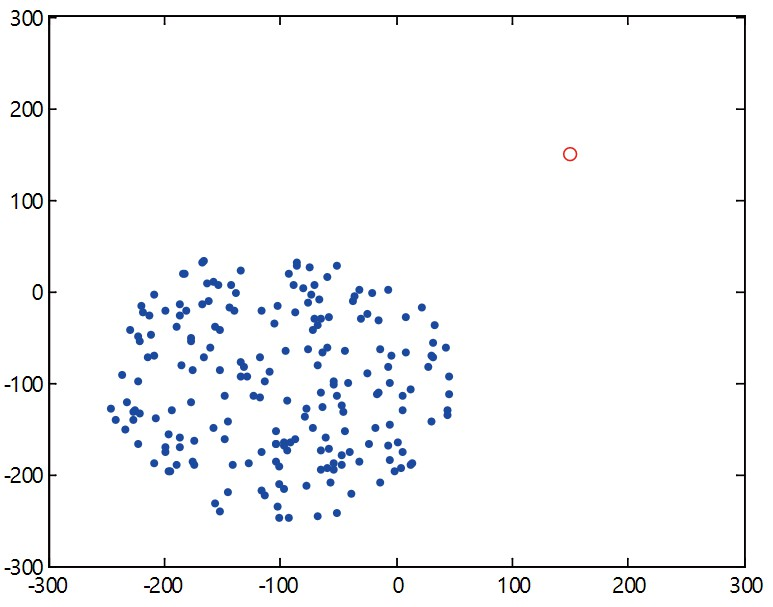# matlab绘制散点图——指定散点落于一个圆形区域内

## matlab代码实现

% 海豚进入前沙丁鱼群的初始状态
N=200;%沙丁鱼群中沙丁鱼的数量
% 沙丁鱼群圆形区域的圆心
x0=-100;
y0=-100;
% 沙丁鱼群圆形区域的实际半径
R=150;
axis tight
hold on
% 沙丁鱼群圆形区域内各个点到圆心的距离
r=R*sqrt(rand(1,N));
% 沙丁鱼群圆形区域内各个点到圆心的方向用seta角表示
seta=2*pi*rand(1,N);
% 沙丁鱼群圆形区域内各个点的的参数方程
x=r.*cos(seta);
y=r.*sin(seta);
% 沙丁鱼群圆形区域内各个点根据圆心坐标移动到指定位置
x=x0+x;
y=y0+y;
% 绘制海豚的圆点
plot(150,150,'rO');
% 绘制沙丁鱼群圆形区域内各个点
plot(x,y,'.');hold on;
% 制定坐标轴的长度
axis([-300 300 -300 300]);
% 坐标轴显示为方框
box on;
% 显示坐标轴
axis on;

## 运行效果